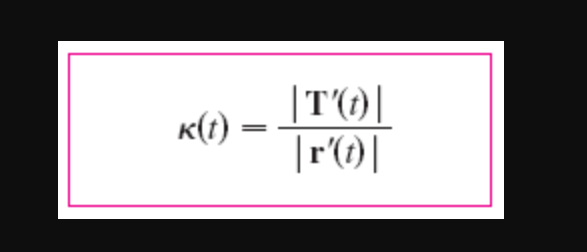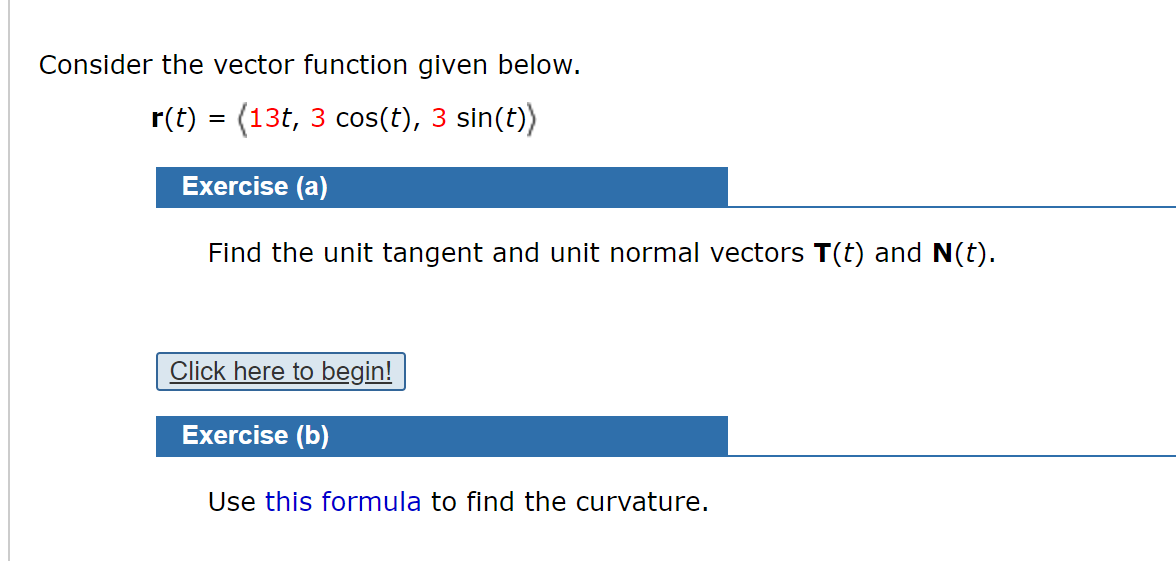# |T)|к() -|r(1) | Consider the vector function given below.r(t) = (13t, 3 cos(t), 3 sin(t))Exercise (a)Find the unit tangent and unit normal vectors T(t) and N(t).Click here to begin!Exercise (b)Use this formula to find the curvature.

Question
2 viewshelp_outlineImage Transcriptionclose|T)| к() - |r(1) | fullscreenhelp_outlineImage TranscriptioncloseConsider the vector function given below. r(t) = (13t, 3 cos(t), 3 sin(t)) Exercise (a) Find the unit tangent and unit normal vectors T(t) and N(t). Click here to begin! Exercise (b) Use this formula to find the curvature. fullscreen
check_circle

Step 1

From the given information It is required to calculate the unit tangent and unit normal vector for the given vector

Step 2

Let r(t) be a differential vector valued function and v(t) = r’(t) be the velocity vector. Then define the unit tangent vector in the direction of the velocity vector as:

Step 3

Now, calculate the v(t) then cal...

### Want to see the full answer?

See Solution

#### Want to see this answer and more?

Solutions are written by subject experts who are available 24/7. Questions are typically answered within 1 hour.*

See Solution
*Response times may vary by subject and question.
Tagged in

### Calculus